# 1. Making your first figure

This tutorial page covers the basics of creating a figure using PyGMT - a Python wrapper for the Generic Mapping Tools (GMT). It will only use the `pygmt.Figure.coast` method for plotting. Later examples will address other PyGMT methods.

## Setting up the development environment

PyGMT can be used in both a Python script and a notebook environment, such as Jupyter. The tutorial’s recommended method is to use a notebook, and the code will be for a notebook environment.

The first step is to import `pygmt`. All methods and figure generation are accessible from the `pygmt` top level package.

```import pygmt
```

## Creating a figure

All figure generation in PyGMT is handled by the `pygmt.Figure` class. Start a new figure by creating an instance of this class:

```fig = pygmt.Figure()
```

To add elements to the figure instance or object (`fig` in this example) different methods can be called on it. This example will use the `pygmt.Figure.coast` method, which can be used to create a map without any other methods or external data. The `pygmt.Figure.coast` method plots the coastlines, borders, and bodies of water using a database that is included in GMT.

First, a region for the figure must be selected. This example will plot some of the coast of Maine in the northeastern US. A Python list can be passed to the `region` parameter with the minimum and maximum X-values (longitude) and the minimum and maximum Y-values (latitude). For this example, the minimum (bottom left) coordinates are (N43.75, W69) and the maximum (top right) coordinates are (N44.75, W68). Negative values can be passed for latitudes in the southern hemisphere or longitudes in the western hemisphere.

In addition to the region, an argument needs to be passed to `pygmt.Figure.coast` to tell it what to plot. In this example, `pygmt.Figure.coast` will be told to plot the shorelines by passing the Boolean value `True` to the `shorelines` parameter. The `shorelines` parameter has other options for finer control, but setting it to `True` uses the default values.

```fig.coast(region=[-69, -68, 43.75, 44.75], shorelines=True)
```

To see the figure, call `pygmt.Figure.show`.

```fig.show()
```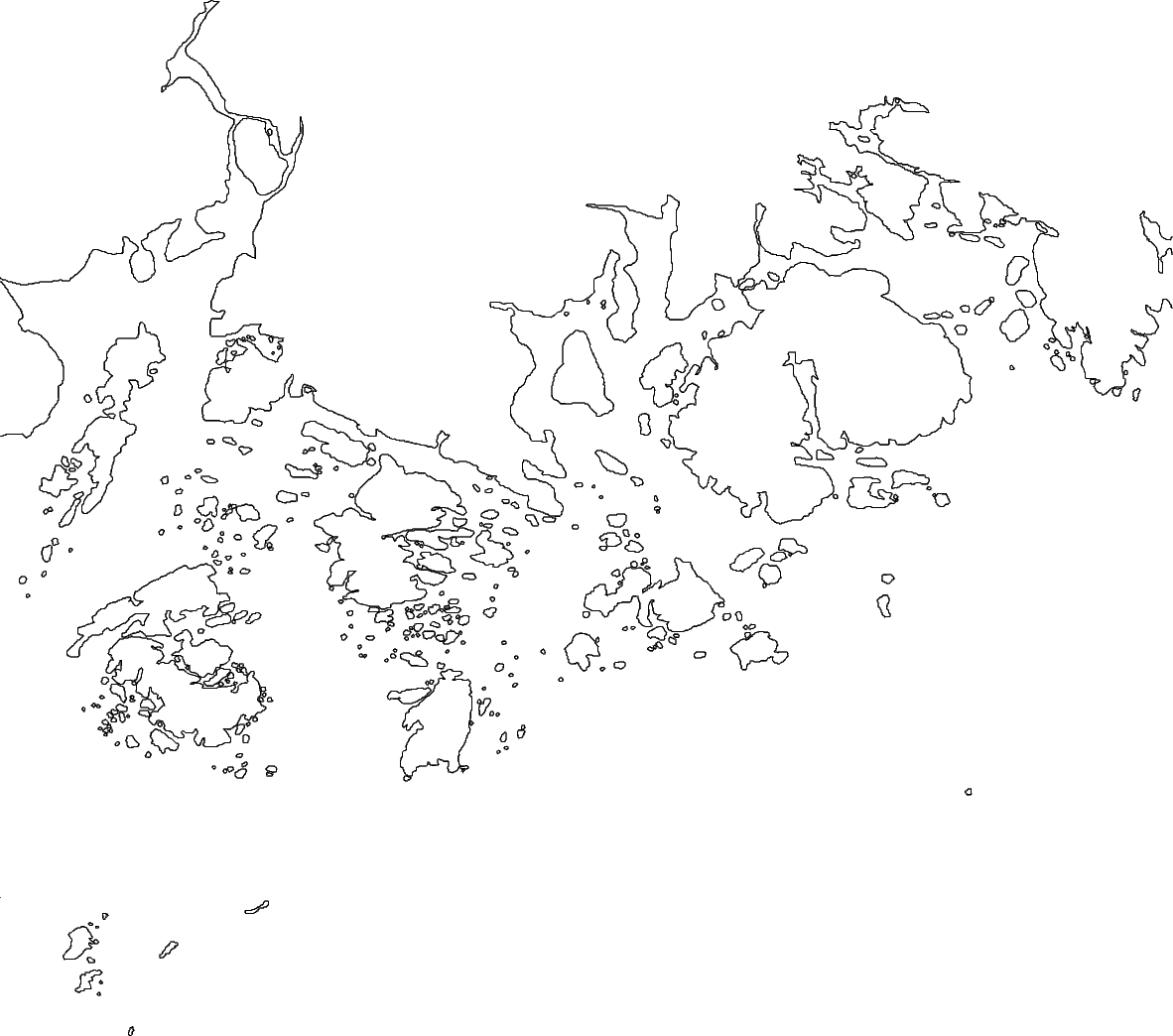## Color the land and water

This figure plots all of the coastlines in the given region, but it does not indicate where the land and water are. Color values can be passed to `land` and `water` to set the colors on the figure.

When plotting colors in PyGMT, there are multiple color codes, that can be used. This includes standard GMT color names (like `"skyblue"`), R/G/B levels (like `"0/0/255"`), a hex value (like `"#333333"`), or a gray level (like `"gray50"`). For this example, GMT color names are used.

```fig = pygmt.Figure()
fig.coast(
region=[-69, -68, 43.75, 44.75],
shorelines=True,
land="lightgreen",
water="lightblue",
)
fig.show()
```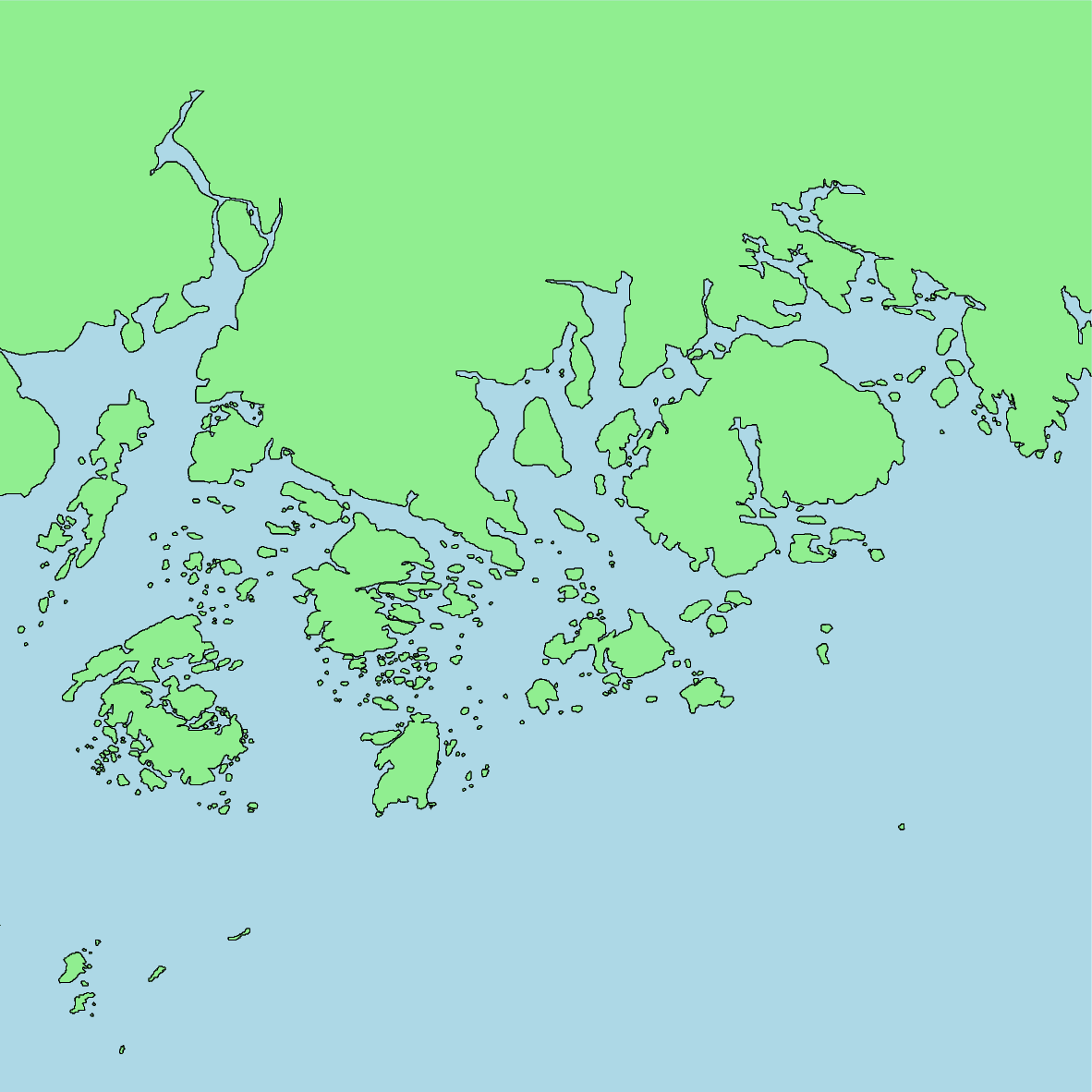## Set the projection

This figure now has its colors set. By default the projection and size of the map is set to `"Q15c+du"`. Here, `"Q"` defines a cylindrical equidistant map projection, `"15c+du"` means setting the maximum (upper) map dimension to 15 cm. However, both of these values can be customized according to the requirements using the `projection` parameter.

The appropriate projection varies for the type of map. The available projections are explained in the projection gallery. For this example, the Mercator projection is set using `"M"`. The width of the figure will be 10 centimeters, as set by `"10c"`. The map size can also be set in inches using “i” (e.g. a 5-inch wide Mercator projection would use `"M5i"`).

```fig = pygmt.Figure()
fig.coast(
region=[-69, -68, 43.75, 44.75],
shorelines=True,
land="lightgreen",
water="lightblue",
projection="M10c",
)
fig.show()
```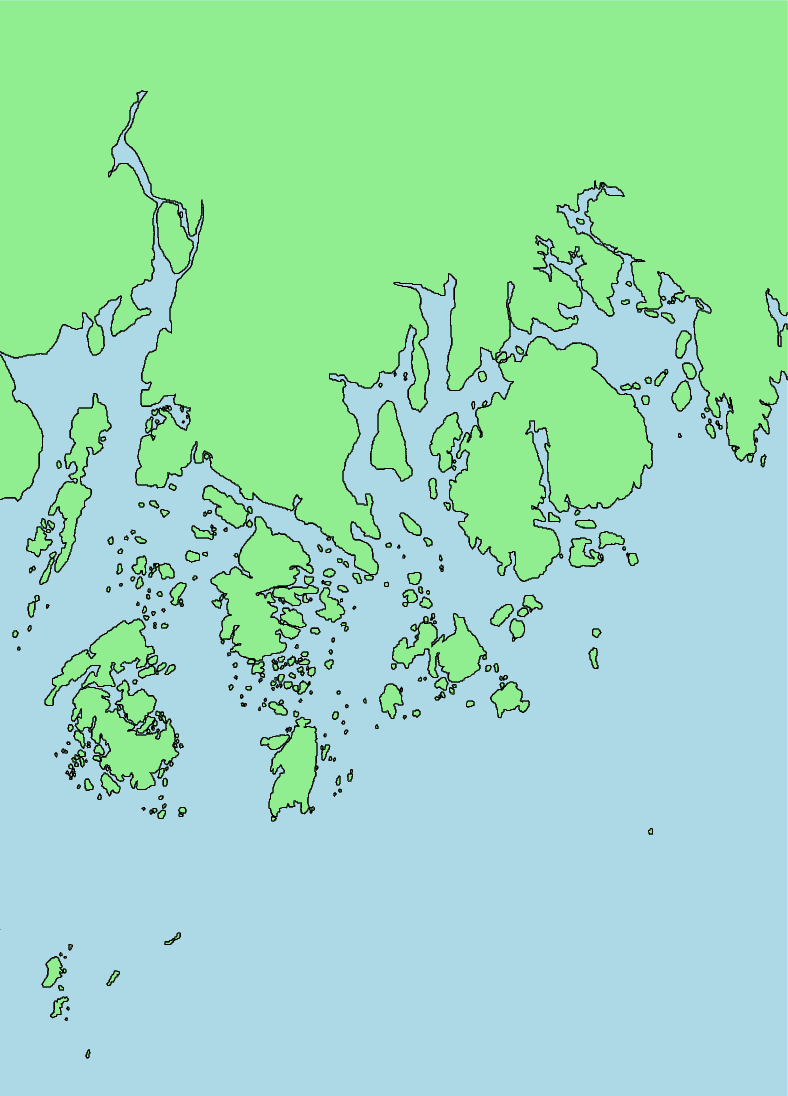While the map’s colors, projection, and size have been set, the region that is being displayed is not apparent. A frame can be added to annotate the latitude and longitude of the region.

The `frame` parameter is used to add a frame to the figure. For now, it will be set to `"a"` to annotate the axes automatically.

```fig = pygmt.Figure()
fig.coast(
region=[-69, -68, 43.75, 44.75],
shorelines=True,
land="lightgreen",
water="lightblue",
projection="M10c",
frame="a",
)
fig.show()
```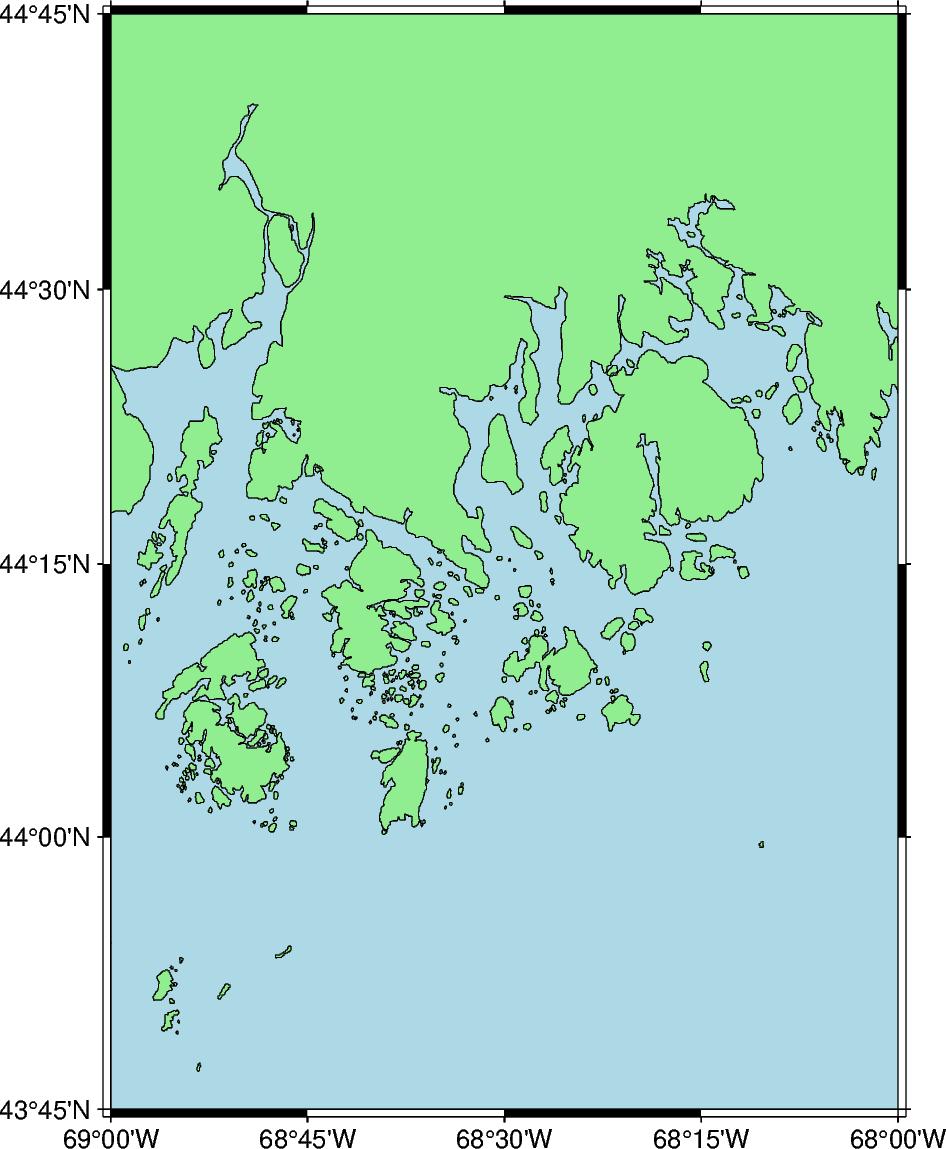The `frame` parameter can be used to add a title to the figure. The title is set by passing `"+t"` followed by the title (e.g. setting the map title to “Title” would be `"+tTitle"`).

To pass multiple arguments to `frame`, a list can be used, as shown in the example below. This format uses `frame` to set both the axes annotations and the figure title.

```fig = pygmt.Figure()
fig.coast(
region=[-69, -68, 43.75, 44.75],
shorelines=True,
land="lightgreen",
water="lightblue",
projection="M10c",
frame=["a", "+tMaine"],
)
fig.show()
```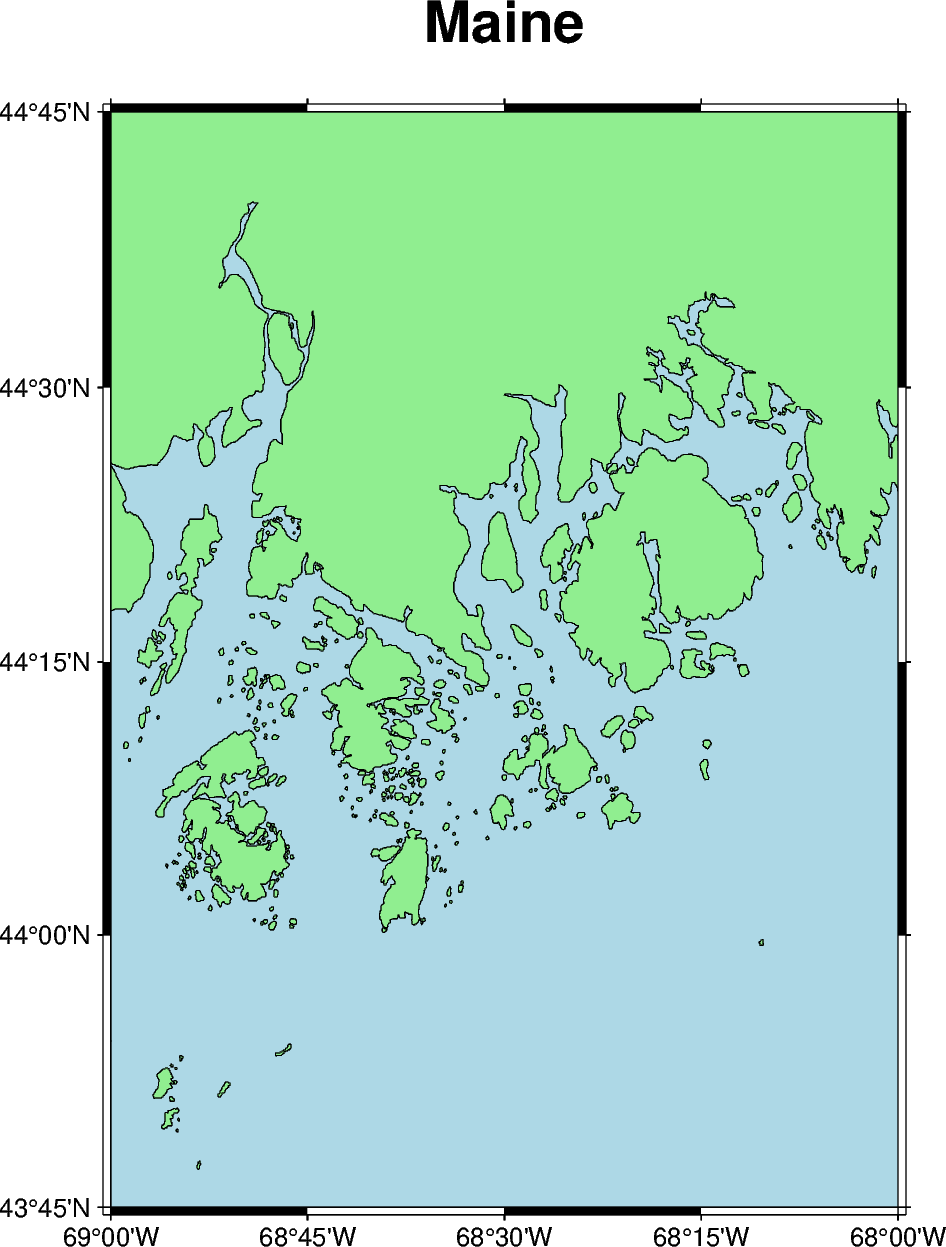This is the end of the first tutorial. Here are some additional exercises for the concepts that were discussed:

1. Make a map of Germany using its ISO country code (“DE”). Pass the ISO code as a Python string to the `region` parameter.

2. Change the color of the landmass to “khaki” and the water to “azure”.

3. Change the color of the lakes (using the `lakes` parameter) to “red”.

4. Create a global map. Set the region to “d” to center the map at the Prime Meridian or “g” to center the map at the International Date Line. When the region is set without using a list full of integers or floating numbers, the argument needs to be passed as a Python string. Create a map with a width of 15 centimeters using the Mollweide (“W”) projection.

Total running time of the script: ( 0 minutes 2.675 seconds)

Gallery generated by Sphinx-Gallery